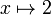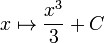# Square function

## Definition

The square function or square map is a function defined on all real numbers that sends any real number to its square, i.e., its product with itself.

## Key data

Item Value
default domain all real numbers, i.e., all of$\R$
range all nonnegative real numbers, i.e.,$[0,\infty)$, same as$\{ y \mid y \ge 0 \}$
no absolute maximum value; absolute minimum value: 0
local maximum values and points of attainment no local maximum value
local minimum values and points of attainment local minimum value of 0 attained at 0
point of inflection (both coordinates) no points of inflection
derivative$x \mapsto 2x$
second derivative$x \mapsto 2$
antiderivative$x \mapsto \frac{x^3}{3} + C$
interval description based on increase/decrease and concave up/down$(-\infty,0)$: decreasing and concave up$(0,\infty)$: increasing and concave up